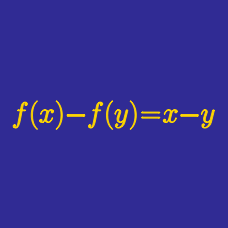Algebra

# Functional Equations - Problem Solving

The function $$f$$ satisfies $(x-y)f(x+y)-(x+y)f(x-y)+4xy(x^2-y^2)=0,$

whenever $$|x| \neq |y |$$.

Given that $$f(1)=2,$$ what is $$f(x)$$?

Function $$f(n)$$ defined over all positive integers $$n$$ satisfies the following: \begin{align} & f(1)=1, f(2)=2, \text{ and}\\ & f(n+2)=f(n+1)+\left(f(n)\right)^2+3581 \text{ for } n \geq 1. \end{align} How many of the $$3581$$ integers $$f(1), f(2), \ldots, f(3581)$$ are multiples of $$7?$$

What is the polynomial $$p(x)$$ that satisfies $p(x^2+4)=\left(p(x)\right)^2+4 \text{ and } p(0)=0?$

A function $$f$$ from the positive integers to the positive integers satisfies the conditions
1) $$f(n) = 2 f(n-1) + 3$$,
2) $$f(1) = 9 .$$

What is the value of $$f(9)$$?

If $$f(x)$$ is a polynomial satisfying $$27 f(x^3) -4f(x^2) - x^6 f(3x) + 46 = 0$$, what is $$f(10)?$$

×

Problem Loading...

Note Loading...

Set Loading...